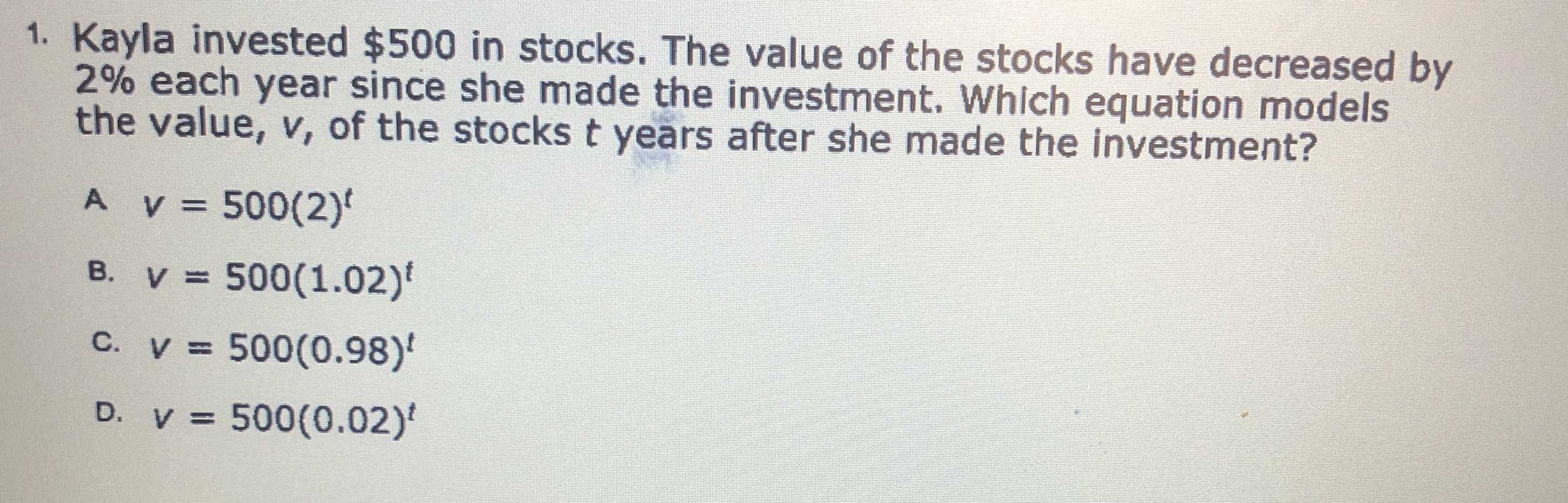### ¿Todavía tienes preguntas de matemáticas?

Pregunte a nuestros tutores expertos
Algebra
Pregunta1. Kayla invested $$\ 500$$ in stocks. The value of the stocks have decreased by $$2 \%$$ each year since she made the investment. Which equation models the value, $$v ,$$ of the stocks $$t$$ years after she made the investment?

A. $$v = 500 ( 2 ) ^ { t }$$

B. $$v = 500 ( 1.02 ) ^ { t }$$

C. $$v = 500 ( 0.98 ) ^ { t }$$

D. $$v = 500 ( 0.02 ) ^ { t }$$